# Test: Mechanical Properties of Solids

## 30 Questions MCQ Test Physics For JEE | Test: Mechanical Properties of Solids

Description
Attempt Test: Mechanical Properties of Solids | 30 questions in 30 minutes | Mock test for NEET preparation | Free important questions MCQ to study Physics For JEE for NEET Exam | Download free PDF with solutions
QUESTION: 1

### A perfectly rigid body is one

Solution:

Explanation:A perfectly rigid body is hypothetical in nature but for some phenomena ( in rotational bodies ) we assume bodies are perfectly rigid i.e. the intermolecular forces are always in equilibrium irrespective of the external forces due to which their shape and size are constant.

QUESTION: 2

### A steel rod 2.0 m long has a cross-sectional area of 0.30 cm2 . It is hung by one end from a support, and a 550-kg milling machine is hung from its other end. Determine the resulting strain. Young's Modulus of Steel (Y) = 20×1010 Pa

Solution:

Stress = F⟂/A = (Mass x Acceleration⟂)/Cross-sectional Area

= (550 kg x 9.8 m/s2)/ (0.30×10−4 m2)

= 1.8×108 Pa

Young's ModulusSteel (Y) = 20×1010 Pa = Stress/Strain

=> Strain = Stress/Y = 1.8×108 Pa / 20×1010 Pa

= 9.0×10−4

QUESTION: 3

### A piece of copper having a rectangular cross-section of 15.2 mm × 19.1 mm is pulled in tension with 44,500 N force, producing only elastic deformation. Calculate the resulting strain? Take Young's modulus of copper as 11 × 1010Pa

Solution:

Given Data,
Length of the piece of copper = l = 19.1 mm = 19.1 × 10-3m
Breadth of the piece of copper = b = 15.2 mm = 15.2× 10-3m
Tension force applied on the piece of cooper, F = 44500N
Area of rectangular cross section of copper piece,
Area = l× b
⇒ Area = (19.1 × 10-3m) × (15.2× 10-3m)
⇒ Area = 2.9 × 10-4 m2
Modulus of elasticity of copper from standard list, η = 42× 109 N/m2
By definition, Modulus of elasticity, η = stress/strain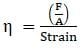⇒ Strain = F/Aη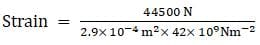⇒ Strain = 3.65 × 10-3
Hence, the resulting strain is 3.65 × 10-3

QUESTION: 4

What diameter should a 10-m-long steel wire have if we do not want it to stretch more than 0.5 cm under a tension of 940 N? Take Young's modulus of steel as 20 × 1010 Pa

Solution:

Y=F x l/A x Δ l
Δ l=0.5cm=0.5x10-2m, l=10M, F=940N
Y=20x1010pa
20x1010=940x10/πr2x0.5x10-10
πr2=94x100/5x10-3x2x1011=94x102/10x108
r2=94/π x 10-7 =2.99 x 10-6
r2 ≅3x10-6
r=1.13x10-10 m
diameter=2r=3.6mm

QUESTION: 5

Elasticity is the property of a body, by virtue of which

Solution:

Explanation:When external force is applied on the solid bodies, the solid bodies get deformed. The atoms or molecules are displaced from their equilibrium positions causing a change in the interatomic ( or intermolicular ) distances. When the deforming foce is removed, the interatomic forces tend to drive them back to their original postions. Thus the body regains its original shape and size.

QUESTION: 6

Elastomers are materials

Solution:

An elastomer is a polymer with viscoelasticity (i. e., both viscosity and elasticity) and very weak intermolecular forces, and generally low Young's modulus and high failure strain compared with other materials. Elastomer rubber compounds are made from five to ten ingredients, each ingredient playing a specific role. Polymer is the main component, and determines heat and chemical resistance, as well as low- temperature performance. Reinforcing filler is used, typically carbon black, for strength properties.

QUESTION: 7

A steel rod 2.0 m long has a cross-sectional area of 0.30 cm2. It is hung by one end from a support, and a 550-kg milling machine is hung from its other end. Determine the elongation. Take Young's modulus of steel as 20 × 1010 Pa

Solution:

σ=Stress and ε=strain
σ=F/A= (550kg) × (9.81m/s2)3×10-5m2/=0.18GPA
ε=Δl/l0=σ/Υ​=0.18×109/200×109​=9×10-4
Δl=εl0= (9×10-4) (2m) = 0.0018m=1.8mm

QUESTION: 8

A 200-kg load is hung on a wire with a length of 4.00 m, a cross-sectional area of 0.200 × 10−4 m2, and a Young’s modulus of 8.00 × 1010N/ m2. What is its increase in length?

Solution: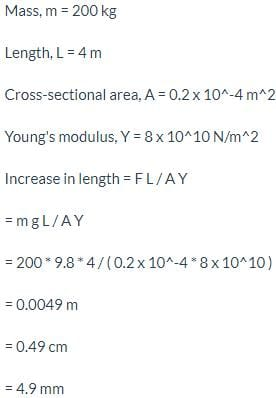QUESTION: 9

The importance of the elastic behavior of materials is

Solution:

Explanation:More the elastic a material is , more it has the property to regain its original position which is required in construction works.

QUESTION: 10

Shear modulus or modulus of rigidity is

Solution:

Shear modulus or modulus of rigidity is the ratio of shearing stress to the corresponding shearing strain, by the definition of shear modulus.

QUESTION: 11

Compute the fractional change in volume of a glass slab, when subjected to a hydraulic pressure of 10 atm. Bulk modulus of glass 37 GPa.

Solution:
QUESTION: 12

If the elastic limit of copper is 1.5 × 108 N/ m2, determine the minimum diameter a copper wire can have under a load of 10.0 kg if its elastic limit is not to be exceeded.

Solution: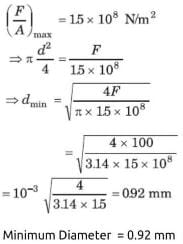QUESTION: 13

When a solid is deformed,

Solution:

Explanation:External force permanently distubed the equilibrium position of the interatomic ( or intermolecular ) forces between the particles of solid bodies.

QUESTION: 14

Rectangular section is rarely used in beams because

Solution:

I section is generally used as a beam because of its high section modulus as its most of the area is situated away from its neutral axis hence it has high moment of inertia i.e. high section modulus i.e. high moment carrying capacity which is the major requirement for a good beam section.

QUESTION: 15

A circular steel wire 2.00 m long must stretch no more than 0.25 cm when a tensile force of 400 N is applied to each end of the wire. What minimum diameter is required for the wire?

Solution: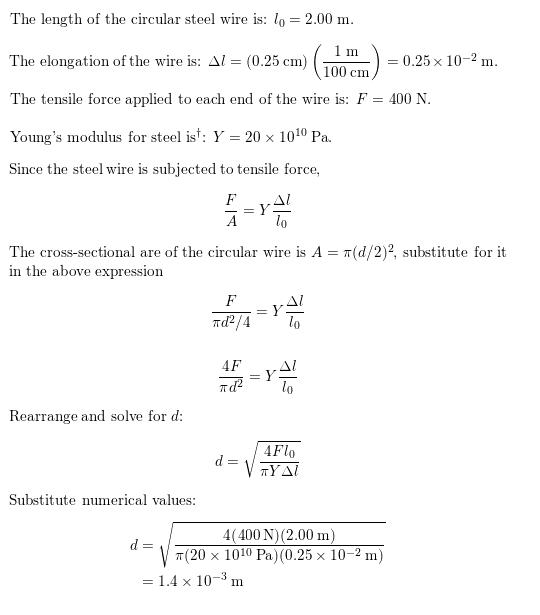QUESTION: 16

A mild steel wire of length 1.0 m and cross-sectional area 0.50 × 10−2 cm2 is stretched, well within its elastic limit, horizontally between two pillars. A mass of 100 g is suspended from the mid-point of the wire. Calculate the depression at the midpoint.

Solution: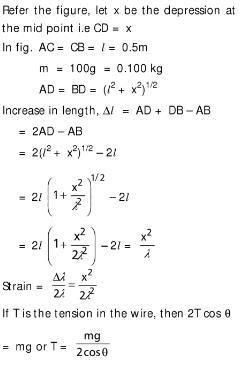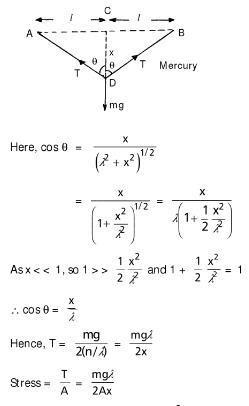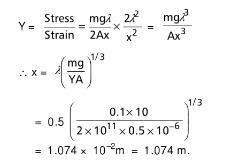QUESTION: 17

You hang a flood lamp from the end of a vertical steel wire. The flood lamp stretches the wire 0.18 mm and the stress is proportional to the strain. How much would it have stretched if the wire were twice as long?

Solution:

F/A = e/L
F/A = 0.18/l ( let the length be l)
F/A = x/2l
0.18/L = x/2l
0.18×2l = xl
0.36l = xl
x = 0.36

QUESTION: 18

Stress is

Solution:
QUESTION: 19

Solution:

Explanation : The load applied to a column would place the column in compression; conversely, a load hanging from a rod would place the rod in tension. Strain is the deformation of a structural member because of stress within the member.

QUESTION: 20

The S.I unit of stress is

Solution:

Stress has its own SI unit called the Pascal. 1 Pascal (Pa) is equal to 1 N/m2. In imperial units stress is measured in pound force per square inch which is often shortened to "psi". The dimension of stress is same as that of pressure.

QUESTION: 21

Material is said to be ductile if

Solution:

A ductile material is one that can withstand a large amount of plastic deformation between the elastic limit and the fracture point.
A material that breaks suddenly when elongated or fracture occurs in it soon after the elastic limit is crossed is called a brittle material.
A ductile material that exhibits extra elongation or deformation and does not fracture is also referred as superplastic material.

QUESTION: 22

In constructing a large mobile, an artist hangs an aluminum sphere of mass 6.0 kg from a vertical steel wire 0.50 m long and 2.5 × 10−3 cm2in cross-sectional area. On the bottom of the sphere he attaches a similar steel wire, from which he hangs a brass cube of mass 10.0 kg. Compute the elongation.

Solution:

Hence 1.6 mm upper, 1.0 mm lower is correct.

QUESTION: 23

volume strain is defined

Solution:
QUESTION: 24

A steel wire of length 4.7 m and cross-sectional area 3.0 × 10−5 m2 stretches by the same amount as a copper wire of length 3.5 m and cross-sectional area of 4.0 × 10−5 m2 under a given load. What is the ratio of the Young’s modulus of steel to that of copper?

Solution: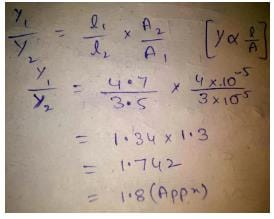QUESTION: 25

A lead cube measures 6.00 cm on each side. The bottom face is held in place by very strong glue to a flat horizontal surface, while a horizontal force F is applied to the upper face parallel to one of the edges. How large must F be to cause the cube to deform by 0.250 mm? (Shear modulus of lead = 0.6 × 1010Pa)

Solution: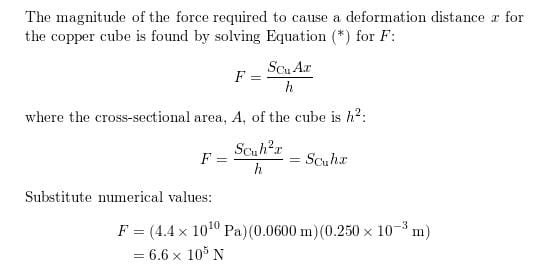QUESTION: 26

Two strips of metal are riveted together at their ends by four rivets, each of diameter 6.0 mm. What is the maximum tension that can be exerted by the riveted strip if the shearing stress on the rivet is not to exceed 6.9 × 107Pa? Assume that each rivet is to carry one quarter of the load.

Solution: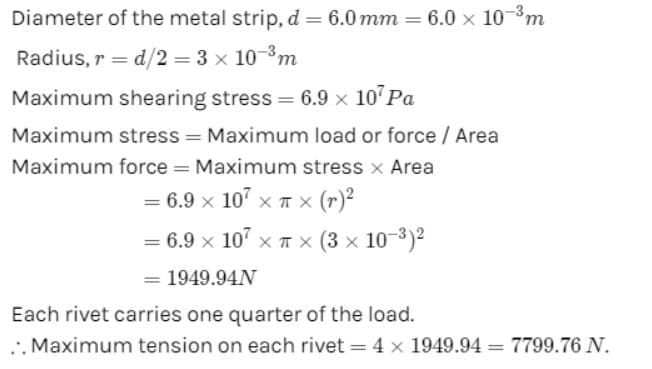QUESTION: 27

According to Hooke’s law

Solution:

Hook’s Law states that for small deformations the stress and strain are proportional to each other.

QUESTION: 28

You hang a flood lamp from the end of a vertical steel wire. The flood lamp stretches the wire 0.18 mm and the stress is proportional to the strain. How much would it have stretched if the wire had the same length but twice the diameter?

Solution:
QUESTION: 29

With reference to figure the elastic zone is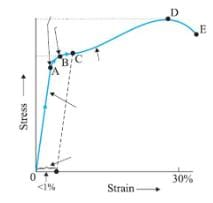Solution:

Hooke’s law: a law stating that the strain in a solid is proportional to the applied stress within the elastic limit of that solid.
In the OA line Hooke’s law is valid because stress is directly proportional to strain.

QUESTION: 30

You hang a flood lamp from the end of a vertical steel wire. The flood lamp stretches the wire 0.18 mm and the stress is proportional to the strain. How much would it have stretched for a copper wire of the original length and diameter?

Solution:Use Code STAYHOME200 and get INR 200 additional OFF Use Coupon Code